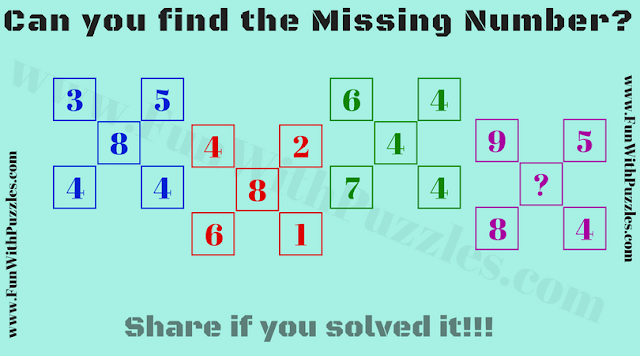This is a very interesting Math Picture Riddle. In this Mathematical Riddle, you are given a group of five numbers. In each of the group, the numbers are related to each other by some Mathematical pattern. Find out this Mathematical pattern and then find the missing number which will replace the question mark. Can you find this missing number?Can you find the missing number?

The answer to this "Math Picture Riddle for Teens", can be viewed by clicking on the button. Please do give your best try before looking at the answer.

Unknown said...